Technical Article

# RTD Signal Conditioning—4-Wire Configuration, Ratiometric Measurement, & Filtering

November 18, 2022 by Dr. Steve Arar

## Learn about RTD (resistance temperature detector) signal conditioning through a four-wire configuration, ratiometric measurement, and input RC filters.

Previously, we explored two-wire and three-wire configurations for both voltage- and current-excited RTD measurements. This article extends the discussion to the four-wire configuration and delves into ratiometric measurement, which is widely used in RTD applications. In addition to that, we’ll go over how RC input filters can be used in ratiometric configurations and learn how matched input and reference path filters can improve the noise performance of a ratiometric configuration.

### RTD 4-Wire Configuration—Voltage Drop and Kelvin Sensing

Figure 1 below shows a four-wire wiring technique for a current-excited RTD.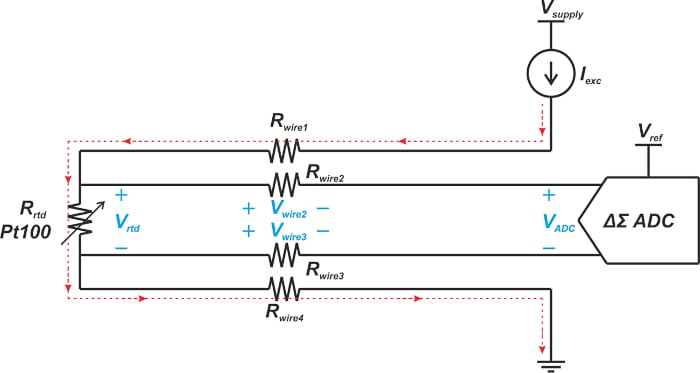##### Figure 1. Block diagram of a four-wire technique in a current-excited RTD.

The analog-to-digital converter (ADC) inputs are high impedance, which causes the excitation current flows through Rwire1, Rrtd, and Rwire4. Since no current flows through Rwire2 and Rwire3, no voltage drops across these two resistors, and the ADC can accurately measure the RTD voltage Vrtd.

While a three-wire configuration requires two matched current sources to eliminate the wire resistance error, the four-wire configuration can achieve this with a single current source. Note that the above method, also called Kelvin sensing, is a general resistance measurement technique that finds use in many other areas, such as resistive current sensing applications.

The four-wire measurement concept can also be applied to a voltage-excited RTD, as illustrated in Figure 2.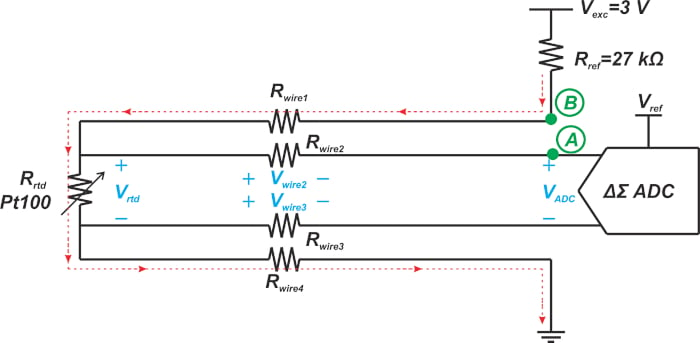##### Figure 2. A block diagram showing the four-wire measurement concept in a voltage-excited RTD.

Again, no voltage drops across Rwire2 and Rwire3, and the ADC accurately measures the voltage across the RTD Vrtd. In a voltage-excited system, the excitation voltage Vexc is known. However, it is impossible to determine the RTD resistance by knowing Vrtd and Vexc because some unknown voltages also drop across Rwire1 and Rwire4. To combat this problem, we can make an extra measurement at a node, such as node B in the above diagram, to figure out the current flowing through the sensor. This is similar to the method we used when discussing the voltage-excited three-wire configuration in the previous article.

Note that with the current excitation, a second measurement is not required because the current flowing through the sensor, Iexc, is already known. The current excitation method is a more straightforward implementation, especially when the wire resistance error is an issue.

### Basics of Ratiometric Measurement

All RTD measurement circuits require an accurate and stable excitation source, as the RTD voltage is a function of the excitation source. For example, consider the circuit diagram in Figure 1. The voltage measured by the ADC relates to the RTD resistance by the following equation:

$V_{ADC}=R_{rtd}\times I_{exc}$

If the excitation current is noisy or drifts with temperature or time, the voltage across the RTD changes even when the temperature is fixed. To maintain high accuracy, the designer needs to use precision components to minimize variations in Iexc

Alternatively, you could use ratiometric measurement. Rather than minimizing the excitation source variations, ratiometric measurement changes the circuit so that the output becomes proportional to the ratio of Iexc to another current (or voltage) in the system.

Let's assume that the circuit is modified in a way that the output equation is changed to:

$V_{ADC}=R_{rtd}\times\frac{I_{exc}}{I_{x}}$

Where Ix is a current in the circuit. Also, if we derive Ix from Iexc in a way that they both experience the same variation, the ratio $$\frac{I_{exc}}{I_{x}}$$ can be kept constant. This makes the measurement system insensitive to the excitation source variations.

In the next section, we’ll see that ratiometric measurements can usually be implemented inexpensively. This inexpensive implementation allows us to use ratiometric configurations to improve accuracy and relax the requirements of certain components, such as the excitation voltage or current source.

### Ratiometric RTD Measurement

Figure 3 shows how the four-wire current-excited measurement can be modified to have a ratiometric configuration.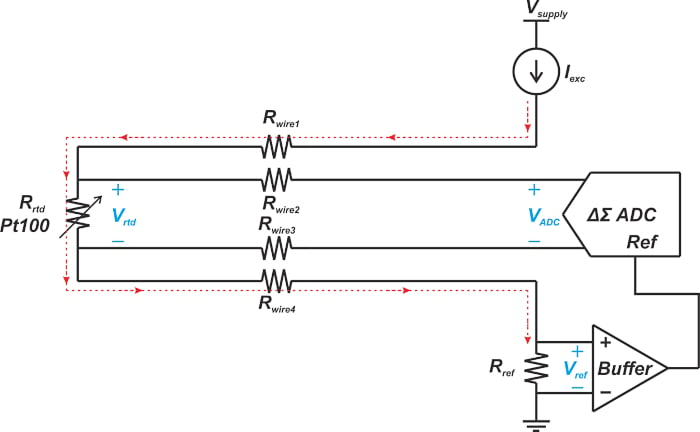##### Figure 3. Block diagram showing the four-wire current-excited measurement can be modified to have a ratiometric configuration.

In this case, the excitation current is passed through a precision reference resistor Rref to create the ADC reference voltage. A buffer is used to sense the voltage across Rref without causing any loading effect on this resistor. Although the buffer is shown as an external component, it is normally integrated into the ADC chip, and an external buffer is not required.

From here, let’s see how the above circuit can produce a ratiometric measurement. The ADC input voltage and reference voltage are given by the following equations:

$V_{ADC}=R_{rtd}\times I_{exc}$

##### Equation 1.

$V_{ref}=R_{ref}\times I_{exc}$

##### Equation 2.

The digital output produced by an n-bit ADC can be typically described by the following equation:

$Digital\,Value=\frac{Analog\,Input\,Voltage}{ADC\,Reference\,Voltage}\times{\Big(}2^{n}-1{\Big)}$

The ADC output is proportional to the ratio of the input voltage to its reference voltage. Substituting Equations 1 and 2 into the above equation, we obtain:

$Digital\,Value=\frac{R_{rtd}\times I_{exc}}{R_{ref}\times I_{exc}}\times{\Big(}2^{n}-1{\Big)}$

This simplifies to:

$Digital\,Value=\frac{R_{rtd}}{R_{ref}}\times{\Big(}2^{n}-1{\Big)}$

The ADC output is no longer a function of the excitation current. However, Rref should be a low tolerance and low drift resistor since any unwanted variation in Rref directly translates into an error in the measurement result. Figure 4 shows the ratiometric configuration for a three-wire RTD application.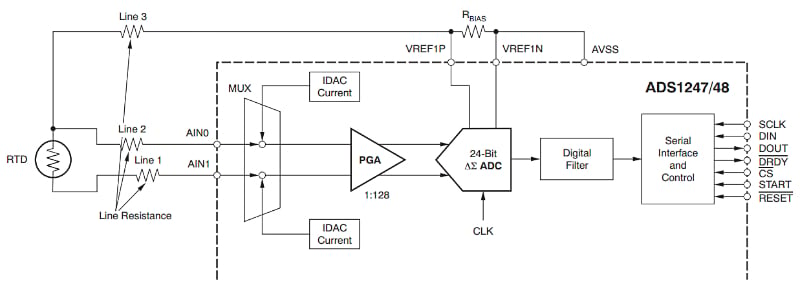##### Figure 4. Example ratiometric configuration of a three-wire RTD application. Image used courtesy of TI

The ratiometric measurement concept can also be applied to a voltage-excited RTD. An example is shown in Figure 5.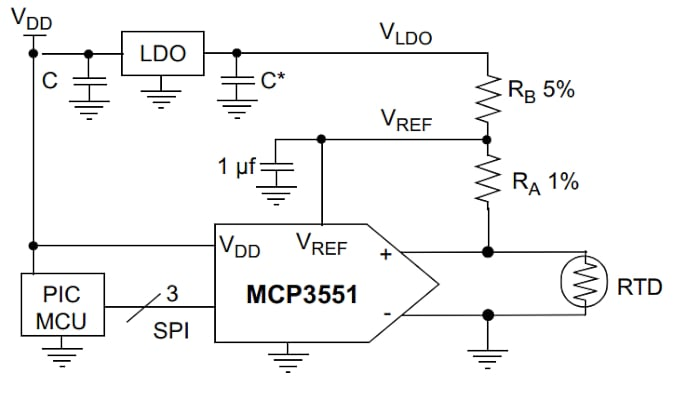##### Figure 5. An example ratiometric measurement block diagram of a voltage-excited RTD. Image used courtesy of Microchip

The above diagram uses the same voltage as the ADC reference voltage and the RTD excitation signal.

### Using an RC Low Pass Filter in Ratiometric Configurations

To attenuate the noise from the excitation current and the environment, RC low-pass filters are placed at the ADC input and reference paths of a ratiometric system. This is illustrated in Figure 6.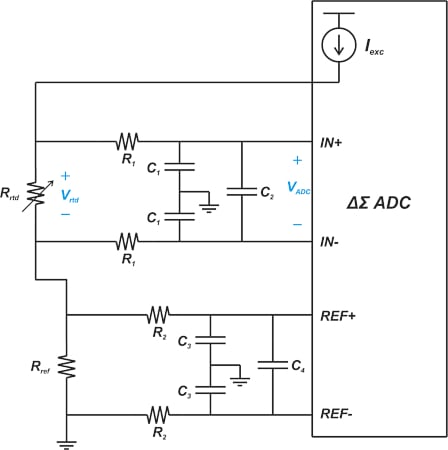##### Figure 6. Using an RC low-pass filter at the ADC input and reference paths of a ratiometric system.

The ratiometric circuit can work without the use of external RC filters; however, the addition of low-pass RC filters can improve the circuit's immunity to radio frequency interference (RFI) and electromagnetic interference (EMI). The filter response for a common-mode noise can be understood by examining the following circuit diagrams in Figures 7a and 7b.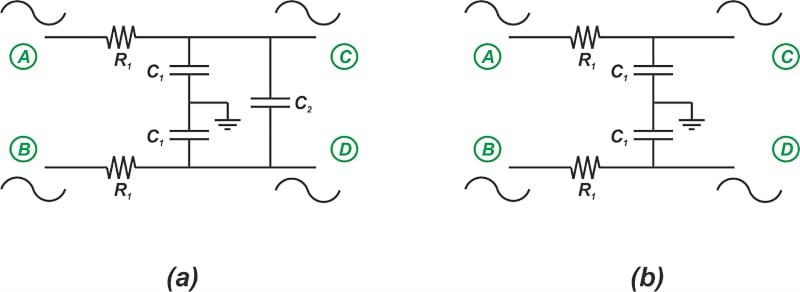##### Figure 7. Example diagrams showing the filter response for common-mode noise.

As shown in Figure 7(a), with a common-mode input, the nodes C and D have the same potential. Thus, no current flows through C2, and this capacitor can be removed from the circuit model. This means that the C1 capacitors determine the common-mode cut-off frequency, which leads to Equation 3:

$f=\frac{1}{2 \pi R_1 C_1}$

##### Equation 3.

On the other hand, for a differential input, C2 can be replaced by a series connection of two 2C2 capacitors, as shown in Figure 8(b).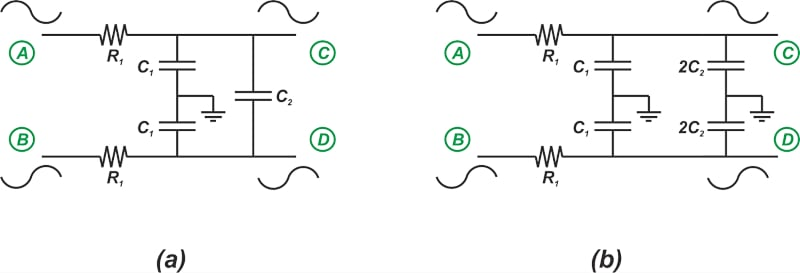##### Figure 8. Example series connection diagrams.

Therefore, the differential cut-off frequency can be expressed as:

$f=\frac{1}{2 \pi R_1 \big ( C_1 + 2C_2 \big)}$

##### Equation 4.

Alternatively, Figure 7(b) shows that the common-mode cut-off frequency for nodes C and D are determined by the upper and lower C1 capacitors, respectively. A mismatch between these two capacitors can lead to a mismatch between the cut-off frequencies of the two paths. Through unequal attenuation of these two filters, the common-mode noise can produce a differential noise at the filter output, which is not at all desired.

To suppress the differential noise produced by mismatched common-mode capacitors, it is recommended that the differential capacitor C2 be at least 10x greater than the common-mode capacitor C1. In other words, the differential capacitor reduces both common-mode and differential noise components.

Several trade-offs should be considered when designing these simple RC filters. A thorough discussion on selecting the filter components to balance these trade-offs is not the goal of this article. However, an important point regarding ratiometric measurements needs to be highlighted: the effect of filter matching on the noise performance of a ratiometric system.

### Matched Filtering to Improve Noise Performance

In the previous section, we discussed that the mismatch of the C1 capacitors within each filter can cause problems (and hence, we added a differential capacitor to each filter). What about the mismatch between the input and reference path filters? To answer this question, note that the ratiometric system tries to make the measurement insensitive to the excitation source variations. This is achieved only if the excitation source variations have the same effect at the ADC analog inputs (IN+ and IN-) and reference inputs (REF+ and REF-). A mismatch between the cut-off frequency of the input and reference paths can lead to unequal attenuation of the excitation noise and reduce the effectiveness of the ratiometric configuration.

The remaining question is: what component values assure that the filters have the same cut-off frequency? Based on Equations 3 and 4, another application note from Analog Devices recommends using the same filters for the input and reference paths. The application note also provides some test results for the circuit diagram shown in Figure 9.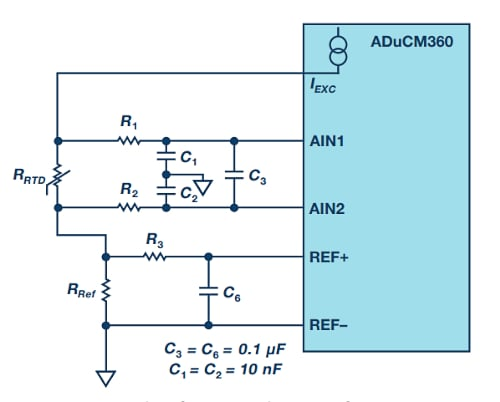##### Figure 9. Example app note diagram. Image used courtesy of Analog Devices

Note that, compared to the general circuit in Figure 6, one resistor and two capacitors are eliminated in the reference path of the above circuit. This is because the REF- pin is connected to the ground in this design. The test results of this circuit are shown in Table 1.

##### Table 1. Data used courtesy of Analog Devices
 ADC Gain ISOURCE (μA) Noise Voltage on 100 Ω Resistor (μV) R1 = R2 = R3 = 1k R1 = R2 = 10k R3 = 1k 16 100 1.6084 1.8395 16 200 1.6311 1.7594 16 300 1.6117 1.9181 16 400 1.6279 1.9292

This test uses a 100 Ω precision resistor instead of the RTD, and the noise voltage at the ADC input pins is measured. The value of RRef is 5.62 kΩ. When the two filters are identical (R= R= R= 1 kΩ), the noise voltage is reduced by about 0.1 µV to 0.3 µV compared to the unmatched case where R= R= 10 kΩ and R= 1 kΩ. In the above example, identical RC filters improve noise performance, but this is not necessarily the maximum achievable noise performance. This will be discussed in the following section.

### Improving Current Source Noise Cancellation

For example, an application note from Texas Instruments discusses that identical filters at the input and reference paths don't produce the maximum cancellation of the current source noise. When deriving Equations 3 and 4, we assumed that a common mode or differential noise appears at the filter inputs (nodes A and B).

This type of analysis is conceptually similar to applying a voltage source to nodes A and B to model the input noise. With this analysis, the effect of the Rrtd and Rref resistors, which is in parallel with the filters, is not considered. These two resistors actually modify the time constant of the RC networks. Since Rrtd and Rref are unequal, identical filters cannot have identical cut-off frequencies. The TI document I mentioned above suggests using the zero-value time constant technique to derive cut-off frequency equations of the two filters.

The zero-value time constant is a method of estimating the bandwidth of a system. For zero-value time constant analysis, the resistance “seen” by each capacitor is determined while the signal source is set to zero (the excitation current is replaced with an open circuit), and the rest of the capacitors are replaced with open circuits. The reason this method is called the zero-value time constant is that all capacitors except the capacitor of interest are set equal to zero to perform the calculation. If the circuit has m capacitors and the resistance seen by a given capacitor Cj is $$R^0_j$$ then the -3 dB bandwidth of the system can be estimated as:

$\omega_{-3dB} =\frac{1}{\sum_{j=1}^{m}R_j^0 C_j}$

##### Equation 5.

For example, to determine the resistance across the C2  and C4 capacitors in Figure 6, we obtain the circuit diagrams in Figure 10(a) and (b), respectively.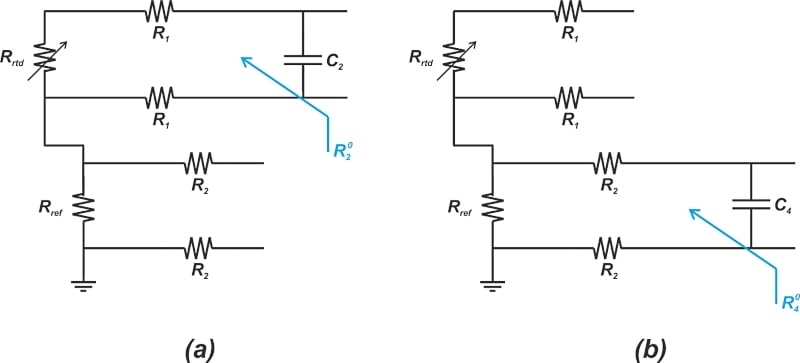##### Figure 10. Diagrams showing the resistance across C2 (a) and C4 (b) capacitors

Equations 6 and 7 show the zero-value time constant (ZVT) associated with C2 and C4, respectively:

${ZVT}_{2}=C_2 \big (2R_1 + R_{rtd} \big)$

##### Equation 6.

${ZVT}_{4}=C_4 \big (2R_2 + R_{ref} \big)$

##### Equation 7.

Originally, the zero-value time constant method was developed to estimate the -3 dB bandwidth of the circuit. To do this, we calculate the time constant of all capacitors in the circuit and then plug them into Equation 5. However, the equation for each individual time constant shows how that particular capacitor interacts with its surrounding resistors to contribute to the circuit bandwidth.

Returning to our RTD measurement system, the input and reference paths will have identical bandwidths if the zero-value time constant of the three capacitors is the same. Therefore, ZVT= ZVT4, which leads to the following equation:

$C_2 \big (2R_1 + R_{rtd} \big)=C_4 \big (2R_2 + R_{ref} \big)$

##### Equation 8.

If C= C4, then the R1 and R2 resistors should be chosen appropriately to yield the same time constant. Based on the above discussion, the TI application note suggests the following example diagram in Figure 11.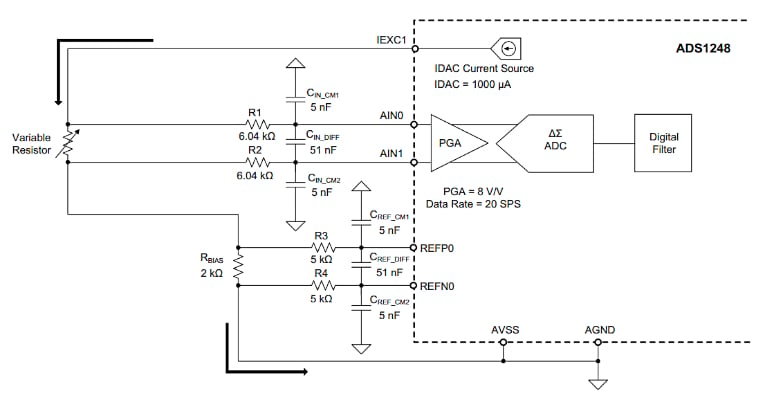##### Figure 11. Example block diagram of ADS1248. Image used courtesy of TI

The sensor resistance is assumed to change from 0 to 250 Ω. Since the variation in the sensor resistance changes the circuit time constant (Equation 6), relatively large resistors are used for the input filter (R= R= 6.04 kΩ). This makes the effect of the RTD variation on the frequency response of the input filter insignificant.

According to Analog Devices’ article, the resistors used in the reference path should be 6.04 kΩ. However, the TI design suggests using 5 kΩ resistors to match the bandwidth of the two filters. Figure 12 shows how the input-referred noise of the system changes with the input voltage level (i.e. the voltage across the RTD).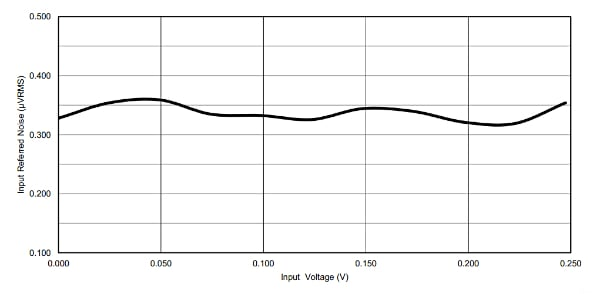##### Figure 12. Graph showing the input-referred noise vs input voltage. Image used courtesy of TI

As you can see, the input-referred noise of the system is about 0.35 µVrms. The input-referred noise of the employed ADC (ADS1248) is typically 0.34 µVrms when the device is configured with a PGA gain of 8 V/V with a data rate of 20 SPS. Additionally, the system noise is close to the reported noise performance of the ADC. Note that when the input and reference path filters are not matched, the input-referred noise of the system can increase with the input signal level to values much higher than that of the ADC. Please refer to the above TI document for more information.

As a final note, it’s worthwhile to mention that the design in Figure 11 only matches the zero-value time constant of the differential capacitors (CIN_DIFF and CREF_DIFF). The time constant of the common-mode capacitors is not exactly the same. However, since the differential-mode capacitors are 10x larger than the common-mode capacitors, it seems that matching the time constant of the differential-mode capacitors has a greater impact on the frequency response of the filters.

Featured image used courtesy of Adobe Stock